• 遇到个数值 分析中的问题，给定一些离散点，求插值出的点，比如， 我传入30个给定点的坐标，然后我要100个插值点，并要求得这100个插值点处 的一阶导数，求C/C++代码，最好是QT平台的c c++
• 三次均匀B样条插值计算 三次均匀B样条插值计算 三次均匀B样条插值计算 三次均匀B样条插值计算
• (yuanjian)三次B样条插值（pudn）(yuanjian)三次B样条插值（pudn）
• 该文档讲述了B样条插值在图像处理中的应用，内容翔实，可供参考。
• 提出了三次B样条插值曲面及其实现的新方法，扩展了三次B样条插值的应用范围
• 目录概述三次b样条插值python实现 概述 三次样条插值（Cubic Spline Interpolation）简称Spline插值，是通过一系列形值点的一条光滑曲线，数学上通过求解三弯矩方程组得出曲线函数组的过程。B样条的定义就不赘述了：...
目录概述三次b样条插值python实现
概述
三次样条插值（Cubic Spline Interpolation）简称Spline插值，是通过一系列形值点的一条光滑曲线，数学上通过求解三弯矩方程组得出曲线函数组的过程。B样条的定义就不赘述了：http://blog.csdn.net/tuqu/article/details/4749586
三次b样条插值python实现
import numpy as np
from numpy.lib.function_base import average
from scipy import interpolate
import matplotlib.pyplot as plt

x = [1,3,5,7,9,11,13,15,17,19,21,23]
y = [6.15,6.65,5.83,11.5,13.61,10.29,9.98,10.28,10.16,8.1,6.9,9.62]

tck,u = interpolate.splprep([x,y],k=3,s=0)
num = 1000
u=np.linspace(0,1,num,endpoint=False)
out = interpolate.splev(u,tck)

plt.plot(x, y, 'ro', out, out, 'b')
plt.legend(['Points', 'Interpolated B-spline', 'True'],loc='best')

plt.show()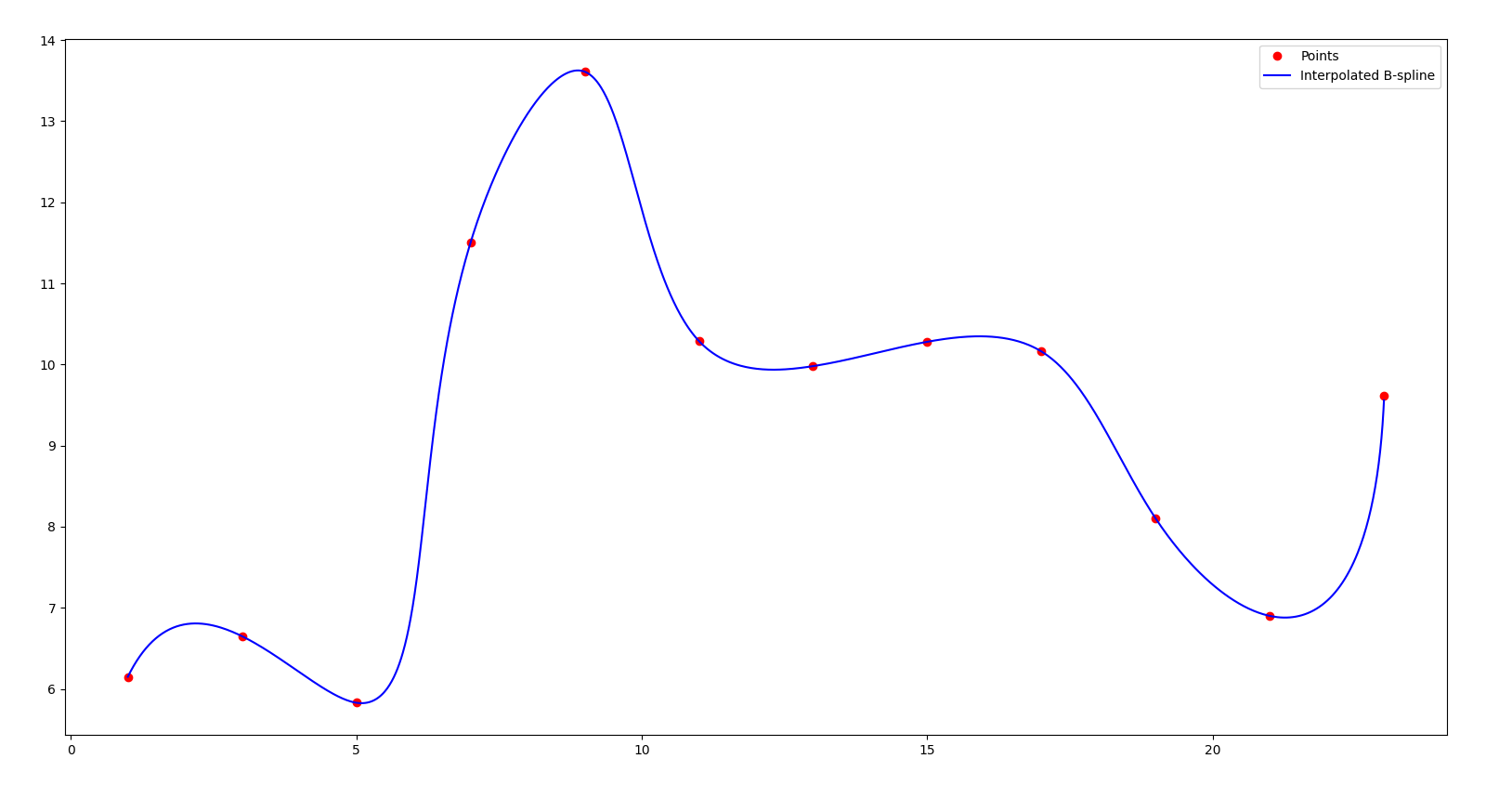展开全文python
• C2连续的三次B样条插值 C2连续的三次B样条插值 C2连续的三次B样条插值
• 三次B样条插值及其误差分析，王少慧，曹艳华，本文从B样条曲线的定义出发，根据已知的型值点，采用均匀参数化法构造节点矢量，从而得到B样条基的具体表达形式；利用逼近思想构�
•研究论文
• 三次均匀有理B样条插值曲线拟合原创-myselfB.m 三次均匀有理B样条插值拟合 通过四重节点办法算出控制点坐标，根据控制点拟合通过型值点的3次B样条曲线matlab
• 如题，在研究基于b样条的图像插值方法，查了很久没找到双三次b样条插值的代码，偏偏很多论文里用到的就是双三次b样条插值。自我理解双三次b样条插值就是用b样条基函数的双三次插值，但b样条基函数又有很多不一样的，...开发语言
• 为此提出了一种基于参数域映射及B样条插值的三维重构方法。采用B样条插值技术对图像进行放大处理,通过像素的参数域映射减小图像的失真及高频信息的丢失,根据放大后图像的灰度信息重构物体的三维表面模型。实验表明,...
• B样条插值的现成matlab程序，3次均匀B样条插值函数matlab程序
• 很多人都想要的B样条插值程序,VC++界面写的，一看就会。。
• 三次B样条插值可用于机器人轨迹规划中速度、加速度、加加速度的实现
• 好多有关B样条插值的博客写的只是部分内容，一上来就是基函数，没有写整个pipeline，一开始看的我是一脸懵逼，我大概梳理一下开发过程，细节不讲，注意这个博客只针对开发需求做B样条插值，是插值！ 1. 开发需求： ...
好多有关B样条插值的博客写的只是部分内容，一上来就是基函数，没有写整个pipeline，一开始看的我是一脸懵逼，我大概梳理一下开发过程，细节不讲，注意这个博客只针对开发需求做B样条插值，是插值！

1. 开发需求：

基于PCL的数据结构对无序点云自动排序；

基于PCL进行3维线条形点云插值，要求穿过每个线条形点云的三维点；

2. B样条插值种类：

2.1 分段贝塞尔曲线：B样条和NURBS的基础；

2.2 均匀B样条；

2.3 准均匀B样条：首尾节点阶数+1的样条；

2.4 非均匀有理B样条曲线（NURBS）：高阶玩家发明的B样条，我也不会；

建议看下大神的博客，见参考文献1

3. pipeline：

3.1 计算控制点[P0,..,Pn]，见参考文献2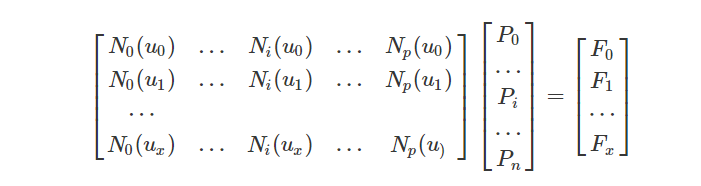3.1.1 求[u0,...,un]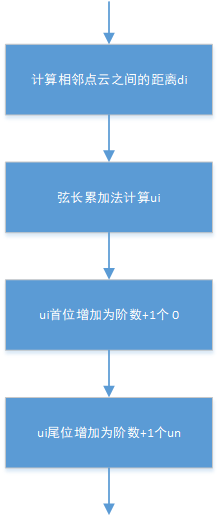3.1.2 求N矩阵

通过基函数和边界求导（矩阵欠定义了，根据三点估算导数/bessel tangent）把N矩阵填好

3.1.3 求F矩阵

源点云和边界求导填入F矩阵

3.1.4 求控制点

最后把控制点求出来

3.2 求插值点

有了控制点Pi和节点列表ui,自己要有一个插值点列表(我的插值点是3.1.1求的Un除以某个用户输入的点数要求,即准均匀B样条插值)，首先判断插值点列表的插值点在节点列表的哪个位置，然后带到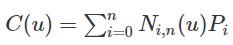求解C(k)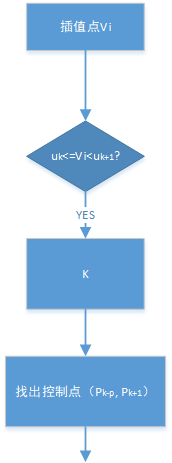参考文献

Fun with geometry: http://www.whudj.cn/?p=450

Fun with geometry:  http://www.whudj.cn/?p=623

展开全文• 12. Scipy Tutorial-一元样条插值前边使用过线性插值linear、最邻近插值nearest以及三次插值cubic，这些插值凸点点多，不够光滑，本章研究样条插值spline,实现尽量让插值函数的曲线显得更光滑(收敛性)一些。样条插值...
12. Scipy Tutorial-一元样条插值前边使用过线性插值linear、最邻近插值nearest以及三次插值cubic，这些插值凸点点多，不够光滑，本章研究样条插值spline,实现尽量让插值函数的曲线显得更光滑(收敛性)一些。样条插值是使用一种名为样条的特殊分段多项式进行插值的形式。由于样条插值可以使用低阶多项式样条实现较小的插值误差，这样就避免了使用高阶多项式所出现的龙格现象，所以样条插值得到了流行。scipy.interpolate包里提供了两个函数splev和splrep共同完成(B-样条:贝兹曲线(又称贝塞尔曲线))插值，和之前一元插值一步就能完成不同，样条插值需要两步完成，第一步先用splrep计算出b样条曲线的参数tck，第二步在第一步的基础上用splev计算出各取样点的插值结果。import numpy as npimport matplotlib.pyplot as pltfrom scipy.interpolate import splev, splrepdef f(x):return x ** 2 + 10 * np.sin(x) + 1x = np.linspace(0, 10, 20)y = f(x)plt.plot(x, y, '*-')plt.show()x2 = np.linspace(0, 10, 200)spl = splrep(x, y, s = 0)y2 = splev(x2, spl)plt.plot(x, y, 'o', x2, y2)plt.show()展开全文• VC++实现的三次B样条插值类，采用Deboor算法，下过很多代码都不是我想要的，花了不少时间实现的。插值点过输入的点，可以修改插值间隔，可以任意等间隔重采样，可以计算切线和法线。此外提供两条优化思路：计算插值...
• F为已知函数，n为从F曲面中取点数目。再用三次B样条插值对这些点进行拟合，拟合结果和实际函数曲面进行比较。
• NURBS-Python (geomdl)是一个跨平台(纯Python)、面向对象的b样条和NURBS库。它与Python版本2.7兼容。它支持有理、无理曲线、曲面、 ...使用geomdl可以方便快速的实现B样条插值/近似拟合： 官方demo代码： from geomdl i
NURBS-Python (geomdl)是一个跨平台(纯Python)、面向对象的b样条和NURBS库。它与Python版本2.7兼容。它支持有理、无理曲线、曲面、
体。NURBS-Python (geomdl)提供了易于使用的数据结构，用于存储几何描述以及基本和高级的评估算法。
官方手册：
使用geomdl可以方便快速的实现B样条插值/近似拟合：
官方demo代码：
from geomdl import fitting
from geomdl.visualization import VisMPL as vis
import numpy as np
import matplotlib.pyplot as plt

# The NURBS Book Ex9.1
points = ((0, 0), (3, 4), (5, 8), (2, 5), (6, 6))
degree = 3  # cubic curve

# Do global curve approximation
curve = fitting.approximate_curve(points, degree)

# Plot the interpolated curve
curve.delta = 0.01
curve.vis = vis.VisCurve2D()
curve.render()

# Prepare points
evalpts = np.array(curve.evalpts)
pts = np.array(points)

# Plot points together on the same graph
fig = plt.figure(figsize=(10, 8), dpi=96)
plt.plot(evalpts[:, 0], evalpts[:, 1])
plt.scatter(pts[:, 0], pts[:, 1], color="red")
plt.show()

运行结果：展开全文python NURBS
• 与之前写的B样条插值差不多 马玉华 2019.11.7 """ import numpy as np from scipy.interpolate import interp1d import matplotlib.pyplot as plt x = np.linspace(0,1,10) # 0-1均匀取10个数 y = np.sin(2*np.pi*x...
• 部分cuda的三维cubic B样条插值代码如下： #ifndef _CUDA_CUBIC3D_H_ #define _CUDA_CUBIC3D_H_ #include "bSplineKernel.cu" //! Tricubic interpolated texture lookup, using unnormalized coordinates. //! ...
• ## 双三次B样条插值

千次阅读 2018-06-27 12:06:00
相对于薄板样条（TPS）的全局形变，B样条（B-spline）对局部形变有很好的支持，因此应用于图像配准领域会得到更好的效果。 插值算法可以理解为对于控制点的加权 ： ...
• 前言：之前写写过一篇B样条曲线，这篇是原文的深度扩展，是针对B样条曲线的一种特殊情况，三次B样条，讨论了其插值和误差分析，添加了一些个人总结。 思路：根据已知的型值点（就是给出的已知的数据点），采用均匀...
• 直方图阈值法因其简单性和抗噪性在图像处理中得到了广泛应用。针对传统模糊熵阈值法对图像分割最佳阈值选取缺乏鲁棒性的问题，提出了参数型模糊熵图像分割新方法。该方法对图像分割最佳阈值选取具有良好的鲁棒性，......